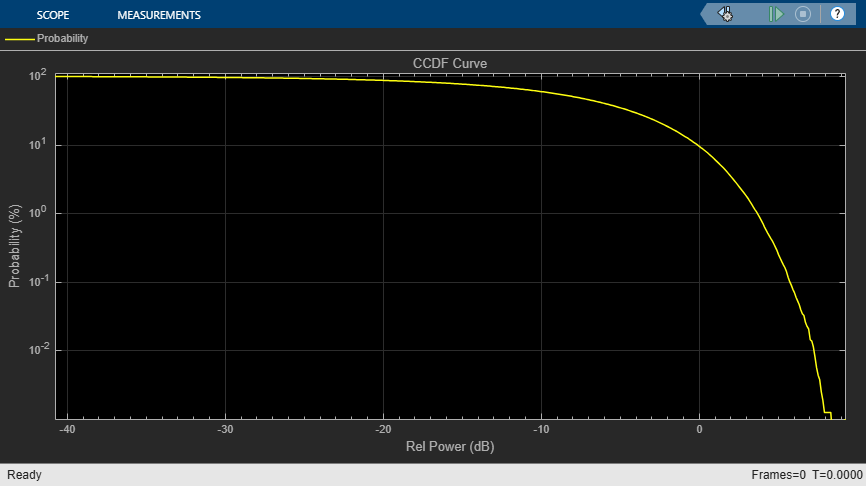# Compute CCDF Measurements of Voltage Signal in Simulink

Compute the cumulative distribution function (CCDF) measurements of a noisy sinusoidal signal using the Power Meter block. These measurements include relative power (dB above the average power) and the probability in percentage.

### Open Model

Open the `CCDFMeasurements.slx` model by clicking the Open Model button.

The input signal is a sum of two sine waves with frequencies set to 1 kHz and 15 kHz, respectively. The frame length and the sample rate of the generated signal is 1024 samples and 8 kHz, respectively. Add zero-mean white Gaussian noise that has a variance of 0.001 to the sinusoidal signal.

The Power Meter block computes the CCDF measurements of the input signal. Set the Measurement parameter to `Average power`, select Compute CCDF, and set CCDF output to `Relative power and probability (whole CCDF curve)`.

The model settings enable the block to measure and output:

• Average power.

• Relative power (in dB): The amount of power by which instantaneous power exceeds the average power of the signal.

• Probability (in percentage): Probability that instantaneous power is above the average power by the relative power value.

For details on how the Power Meter block computes these measurements, see Algorithms.

The Power Meter block automatically sets the length of the sliding window to infinity and the length of the overlap between windows to zero. The block measures power in dBm units. Visualize the CCDF measurements using the Array Plot block.

### Compute CCDF Measurements

Run the model. The Array Plot window displays the CCDF curve which is the plot of the relative power (in dB) against the probability (in percentage). To plot the relative power on the x-axis, set the X-Offset in the Array Plot window to the starting value in the relative power vector. Choose the value of Sample Increment such that the entire relative power vector can be plotted on the x-axis.

To find the X-Offset and the Sample Increment properties, in the Plot Tab of the Array Plot window, click Settings.